# Contact parameters identification of a cannon cradle and its bushing based on FDA and ELM

## Hao Wen1, Baolin Hou2

1, 2School of Mechanical Engineering, Nanjing University of Science and Technology, Nanjing, China

2Corresponding author

Vibroengineering PROCEDIA, Vol. 20, 2018, p. 202-207. https://doi.org/10.21595/vp.2018.20234
Received 7 September 2018; accepted 21 September 2018; published 19 October 2018

34th International Conference on Vibroengineering in Shanghai, China, October 19-21st, 2018

Copyright © 2018 Hao Wen, et al. This is an open access article distributed under the Creative Commons Attribution License, which permits unrestricted use, distribution, and reproduction in any medium, provided the original work is properly cited.
Views 19
CrossRef Citations 0
Abstract.

An identification method based on functional data analysis (FDA) and extreme learning machine (ELM) is presented to identify the contact parameters of a cannon cradle and its bushing. A virtual prototype of the cannon is built in ADAMS. The response curves of muzzle vertical acceleration with different contact parameters of the cradle and its bushing are obtained by simulation experiments and used for FDA as sample data. Features of the sample data are extracted by FDA and functional principle component analysis (FPCA), and the features and contact parameters are used to train the ELM. Simulation data and test data are used to verify the proposed method. The presented method is also proved to be feasible and effective by comparing actual muzzle vertical acceleration curve and the muzzle vertical acceleration curve from the virtual prototype with respect to the test data identification results.

Keywords: parameter identification, functional data analysis, extreme learning machine, cradle.

#### 1. Introduction

A cradle is the carrier of a cannon recoiling part, which rotates around the trunnion and transfers firing load to other carriages. Changes in contact parameters of the cradle and its bushing will affect mechanism kinematic accuracy during firing and firing accuracy of the cannon. To establish a virtual prototype of the cannon, it is significant to infer the contact parameters of the cradle and the front and back bushing. Due to a cannon is a complex and multi-parameter system, determination of key parameters is a core problem in the modeling process, but these parameters are difficult to measure and can only be obtained by identification.

Since it is difficult to find the analytic expressions of the measurable response to the parameters to be identified, optimization method based on analytic expression cannot be used to identify the contact parameters of the cradle and its bushing . Machine learning (ML) can estimate the dependence of data based on the known sample, so ML can predict and judge the unknown data. Actual experiments can obtain high-quality samples, but for a cannon system, a large number of experiments require a lot of cost and resources and it is easier to obtain samples by virtual simulation.

In this paper, a virtual prototype of the cannon is established, and the response curves of muzzle vertical acceleration are obtained by sampling and simulation experiments of the parameters to be identified and used for FDA as sample data. Using FDA and FPCA to extract the sample data, the extracted features and parameters to be identified are used for ELM training as training samples. Finally, the simulation data and test data are used to identify the parameters and the presented method is proved to be feasible and effective by comparing real muzzle vertical acceleration curve and the muzzle vertical acceleration curve from the virtual prototype with respect to the test data identification results.

#### 2. Modeling and simulation of virtual prototype

The recoiling part of a cannon on zero firing angle is mainly affected by recoil brake force, recuperator force, sealing device friction and cradle rail friction. According to the topological structure of the cannon, the dynamics model is established in ADAMS as shown in Fig. 1.

According to the research purpose, the contact parameters to be identified of the cradle and its bushing are the following five parameters: contact stiffness ${K}_{f}$ and ${K}_{r}$, damping $C$, and clearance ${D}_{f}$ and ${D}_{r}$. Consulting engineering experience, the range of ${K}_{f}$ is [100000, 200000]N⋅mm, the range of ${K}_{r}$ is [150000, 300000] N⋅mm, the range of $C$ is [10, 50], and the range of ${D}_{f}$ and ${D}_{r}$ is [0.5, 1.5] mm. The five parameters are sampled within the distribution range, and virtual simulation experiments are carried out according to the sampling results. A total of 100 groups of muzzle vertical acceleration curves in counter-recoiling movement are obtained as shown in Fig. 2.

Fig. 1. Virtual prototype of the cannon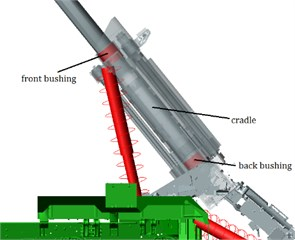Fig. 2. Sample curves of muzzle vertical acceleration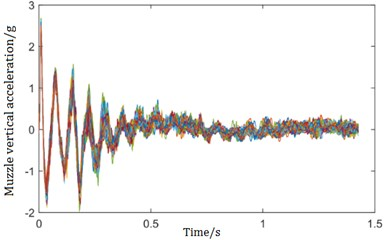#### 3.1. The FDA and FPCA algorithm

The basic idea of FDA is to treat the observed data as a whole, expressed as a smooth curve or a continuous function, and analyze it from a functional perspective. Assume that the $i$th observation sample contains a series of observation values ${y}_{i1}$, ${y}_{i2}$, ..., ${y}_{in}$, it can be transformed to be a function ${x}_{i}\left(t\right)$ by FDA, where $t$ is the argument. Generally, the non-periodic data are expanded by the B-spline basis function system. Its functional form is represented by a linear combination of basis functions by letting $\mathbf{c}$ indicate the vector of length $K$ and $\mathbf{\varphi }$ as the functional vector whose elements are the basis functions ${\varphi }_{k}$:

(1)
$\mathbf{x}={\mathbf{c}}^{\mathrm{Τ}}\mathbf{\varphi }={\mathbf{\varphi }}^{T}\mathbf{c}.$

For making the estimated curves more stable, a roughness penalty $PE{N}_{m}\left(x\right)$ is introduced:

(2)
${\mathrm{P}\mathrm{E}\mathrm{N}}_{m}\left(x\right)=\int {\left[{D}^{m}x\left(s\right)\right]}^{2}ds=\int {\left[{D}^{m}{\mathbf{c}}^{T}\mathbf{\varphi }\left(s\right)\right]}^{2}ds=\int {\mathbf{c}}^{T}{D}^{m}\mathbf{\varphi }\left(s\right){D}^{m}{\mathbf{\varphi }}^{T}\left(s\right)\mathbf{c}ds={\mathbf{c}}^{T}\mathbf{R}\mathbf{c},$

where ${D}^{m}$ is the m-order derivative and $\mathbf{R}=\int {D}^{m}\mathbf{\varphi }\left(s\right){D}^{m}{\mathbf{\varphi }}^{T}\left(s\right)ds$.

Define $\mathbf{H}$ as a weighted matrix and $\lambda$ as a smoothing parameter, we obtain:

(3)
${\mathrm{P}\mathrm{E}\mathrm{N}\mathrm{S}\mathrm{S}\mathrm{E}}_{m}\left(\mathbf{y}\left|\mathbf{c}\right\right)=\left(\mathbf{y}-\mathbf{\Phi }\mathbf{c}{\right)}^{T}\mathbf{H}\left(\mathbf{y}-\mathbf{\Phi }\mathbf{c}\right)+\lambda {\mathbf{c}}^{T}\mathbf{R}\mathbf{c}.$

The expression for the estimated coefficient vector is:

(4)
$\stackrel{^}{\mathbf{c}}=\left({\mathbf{\Phi }}^{T}\mathbf{H}\mathbf{\Phi }+\lambda \mathbf{R}{\right)}^{-1}{\mathbf{\Phi }}^{T}\mathbf{H}\mathbf{y}.$

FPCA is an expansion of Principle Component Analysis to Hilbert space. According to the derivation of Ramsay , the eigenfunction must satisfy the following equation:

(5)
$\int v\left(s,t\right)\xi \left(t\right)dt=\rho \xi \left(s\right),$

where $\rho$ is the eigenvalue and $v\left(s,t\right)=\frac{1}{N}\sum _{i=1}^{N}{x}_{i}\left(s\right){x}_{i}\left(t\right)$ is the covariance function.

Let $\xi$ indicate the eigenfunction and define an integral transform $V$, $V\xi =\int v\left(\cdot ,t\right)\xi \left(t\right)dt$, then Eq. (5) can be expressed as:

(6)
$V\xi =\rho \xi .$

The principle component function also needs to be smoothed. Maximizing the variance of the samples with a roughness penalty:

(7)
$\mathrm{P}\mathrm{C}\mathrm{A}\mathrm{P}\mathrm{S}\mathrm{V}\left(\xi \right)=\frac{var\int \xi {x}_{i}dt}{{‖\xi ‖}^{2}+\lambda ×{\mathrm{P}\mathrm{E}\mathrm{N}}_{2}\left(\xi \right)}.$

For sample function, the form of basis function combination is ${x}_{i}\left(t\right)=\sum _{k=1}^{K}{c}_{ik}{\varphi }_{k}\left(t\right)$. Let $\mathbf{A}$ be the covariance matrix of vectors ${\mathbf{c}}_{i}$ and define $\mathbf{W}=\int \mathbf{\varphi }{\mathbf{\varphi }}^{T}$, then Eq. (7) can be written as:

(8)
$\mathrm{P}\mathrm{C}\mathrm{A}\mathrm{P}\mathrm{S}\mathrm{V}=\frac{{\mathbf{b}}^{T}\mathbf{W}\mathbf{A}\mathbf{W}\mathbf{b}}{{\mathbf{b}}^{T}\mathbf{W}\mathbf{b}+\lambda {\mathbf{b}}^{T}\mathbf{R}\mathbf{b}}.$

The eigenequation corresponding to Eq. (8) is given by:

(9)
$\mathbf{W}\mathbf{A}\mathbf{W}\mathbf{b}=\rho \left(\mathbf{W}+\lambda \mathbf{R}\right)\mathbf{b}.$

Now, preforming a Choleski factorization $\mathbf{W}+\lambda \mathbf{R}=\mathbf{L}{\mathbf{L}}^{T}$ and defining $\mathbf{S}={\mathbf{L}}^{-1}$, the Eq. (9) can be written as:

(10)
$\left(\mathbf{S}\mathbf{W}\mathbf{A}\mathbf{W}{\mathbf{S}}^{T}\right)\left({\mathbf{L}}^{T}\mathbf{b}\right)=\rho \left({\mathbf{L}}^{T}\mathbf{b}\right).$

Defining $\mathbf{u}={\mathbf{L}}^{T}\mathbf{b}$, the Eq. (10) can be written as:

(11)
$\left(\mathbf{S}\mathbf{W}\mathbf{A}\mathbf{W}{\mathbf{S}}^{T}\right)\mathbf{u}=\rho \mathbf{u}.$

This is a eigenvalue problem, and $\mathbf{u}$, $\mathbf{b}$ and the eigenfunction can be carried out in turns.

#### 3.2. Features extraction of muzzle vertical acceleration curves

In this paper, a 4-order B-spline basis function and a 2-order roughness penalty function with smoothing coefficient $\lambda =$ 50000 are used. Fig. 3(a) shows the sample data for the muzzle vertical acceleration after this functional procedure. Fig. 3(b) shows the first ten principal component functions.

To ensure that the sum of ratio of the principle component functions is greater than 90 %, we select the first 10 principal component functions. For every sample, after it is made to be functional data, the principal component scores can be acquired by calculating the inner product of the functional data and principal component functions, and the principal component scores are the eigenvalues we need.

#### 4.1. ELM algorithm

ELM is a typical single-hidden layer feedforward neural network . Assume any independent sample $\left({\mathbf{x}}_{i},{\mathbf{t}}_{i}\right)$, ${\mathbf{x}}_{i}=\left[{x}_{i1},{x}_{i2},...,{x}_{in}{\right]}^{T}\in {\mathbf{R}}^{n}$, ${\mathbf{t}}_{i}=\left[{t}_{i1},{t}_{i2},...,{t}_{im}{\right]}^{T}\in {\mathbf{R}}^{m}$, mathematical model of ELM with $n$ input nodes and $m$ output nodes is:

(12)

where $\stackrel{~}{N}$ is the number of hidden layer nodes, $g\left(x\right)$ is the activation function, ${w}_{i}$ is the weight connectiing the $i$th input node and hidden node, ${\mathbf{\beta }}_{i}$ is the weight connecting the $i$th hidden node and output node, and ${b}_{i}$ is the threshold of the $i$th hidden node.

Fig. 3. The results of FDA and FPCA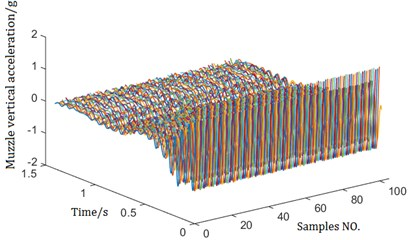a) Muzzle vertical acceleration after function procedure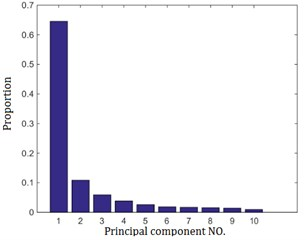b) Proportion of first ten principal components

When the number of hidden nodes is equal to training samples, ELM can approximate the training sample with zero error. There are ${\mathbf{\beta }}_{i}$, ${\mathbf{w}}_{i}$, ${b}_{i}$ and output layer matrix $\mathbf{H}$ which make:

(13)

$\beta$ can be acquired by solving the least squares solution of Eq. (13):

(14)
$\stackrel{^}{\mathbf{\beta }}={\mathbf{H}}^{+}\mathbf{T},$

where ${\mathbf{H}}^{+}$ is the Moore-Penrose generalized inverse of the output matrix.

The identification accuracy and generalization performance of ELM are evaluated by average relative error $E$ and determination coefficient ${R}^{2}$, respectively:

(15)

#### 4.2. Testing of muzzle vertical acceleration

The artificial recoil method is used to test the muzzle vertical acceleration by using the wireless acceleration node A104EX in the wireless test system. Fig. 4 shows the components of the wireless test system. The sensors are installed on the muzzle brake as shown in Fig. 5. The test is repeated 5 times at a firing angle of 0°, and the test results are shown in the Fig. 6.

Fig. 4. Hardware components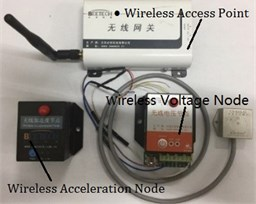Fig. 5. The installation diagram of the sensors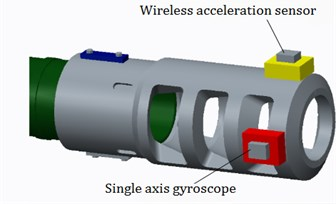Fig. 6. Test curves of muzzle vertical acceleration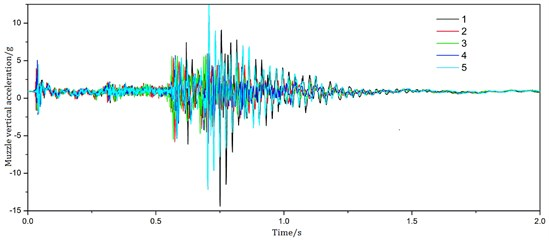#### 4.3. Identification results and analysis

In this paper, 100 groups of sample curves are obtained, of which 95 groups are used for ELM training, and the other 5 groups are used to verify the identification effect of ELM. Table 1 shows the identification results of five contact parameters, and the first five times are the identification results of five groups of simulation data and the last is the identification results of test data. The average relative error $E$ and determination coefficient ${R}^{2}$ of the identification results are shown in Table 2. The identification results of ${E}_{f}$ and ${E}_{b}$ are better and the identification results of ${K}_{f}$ and ${K}_{b}$ can also meet the requirements. The identification results of $C$ are relatively poor, and the probable cause is that $C$ has less effect on the muzzle vertical acceleration, or the number of the samples used to train ELM is insufficient.

Table 1. Identification results

 No. of identification $C$ ${D}_{f}$ (mm) ${D}_{r}$ (mm) ${K}_{f}$ (×105 N⋅mm) ${K}_{r}$ (×105 N⋅mm) 1 Real value 21.2857 0.8438 1.2438 1.7786 2.9587 Identification value 22.3214 0.8306 1.2275 1.7571 2.9852 2 Real value 17.6429 1.1844 1.1281 1.3293 2.9913 Identification value 17.6071 1.1975 1.1206 1.3607 2.8201 3 Real value 24.5357 0.5594 0.9234 1.3929 1.6229 Identification value 27.1286 0.5625 0.9313 1.2857 1.9583 4 Real value 21.6929 0.8825 0.6094 1.3536 2.9737 Identification value 24.2143 0.9281 0.5813 1.3821 2.8256 5 Real value 27.7143 0.9625 1.0063 1.6393 1.5703 Identification value 25.8714 96563 1.0156 1.7071 1.7862 6 Real value \ \ \ \ \ Identification value 14.5357 1.3924 0.8263 2.2214 1.7903

The parameters identified by the test data are substituted into the virtual prototype model for simulation, and the test curve is compared with the simulated curve. As shown in Fig. 7, the two curves are more consistent, which proves the reliability of the identification results.

Table 2. Average relative error and determination coefficient

 Parameter Average relative error Determination coefficient $C$ 8.01 % 0.7681 ${D}_{f}$ 1.40 % 0.9902 ${D}_{r}$ 1.47 % 0.9962 ${K}_{f}$ 3.13 % 0.9035 ${K}_{r}$ 9.12 % 0.9749

Fig. 7. Comparison of identification curve and test curve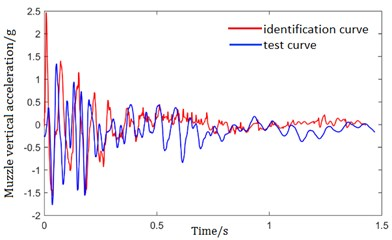#### 5. Conclusions

In this paper, a virtual prototype of a cannon is established in ADAMS. The muzzle vertical acceleration curves are obtained by the sampling of the parameters to be identified and simulation experiments and used for FDA as sample data. Using FDA and FPCA to extract the sample data, the extracted features and parameters to be identified are used for ELM training. The simulation data and test data are used to identify the contact parameters of the cradle and bushing. The presented method is proved to be feasible and effective by comparing real muzzle vertical acceleration curve and the muzzle vertical acceleration curve from the virtual prototype with respect to the test data identification results.

1. Zhao Q. Q., Hou B. L. Parameter identification of a shell transfer arm using FDA and optimized ELM. Chinese Journal of Engineering, Vol. 39, 4, p. 611-618. [Search CrossRef]
2. Zong Weiwei, Huang Guang Bin Face recognition based in extreme learning machine. Neurocomputing, Vol. 74, Issue 16, 2011, p. 2541-2551. [Publisher]
3. Ramsay J. O. When the data are functions. Psychometrika, Vol. 47, Issue 4, 1982, p. 379. [Publisher]
4. Ramsay J. O., Hooker G., Graves S. Functional Data Analysis with R and MATLAB. Second Edition, Springer Science + Business Media Inc., New York, 2005. [Search CrossRef]
5. Li M. Analysis of water data function based on principle component analysis method. Natural Sciences Edition, Journal of Hefei University, Vol. 24, Issue 4, 2014, p. 21-25. [Search CrossRef]
6. Zipunnikov V., Caffo B., Yousem D. M., et al. Functional principal component model for high-dimensional brain imaging. NeuroImage, Vol. 58, Issue 4, 2011, p. 772-784. [Publisher]
7. Gao X. X., Sun H. G., Hou B. L., et al. Optimization design of positioning precision for howitzer shell transfer arm with parameter uncertainty. Acta Armamentarii, Vol. 35, Issue 6, 2014, p. 776-781. [Search CrossRef]
8. Huang G. B., Zhao Q. Y., Siew C. K. Extreme learning machine: theory and applications. Neurocomputing, Vol. 70, Issue 1, 2006, p. 489-501. [Publisher]